Select Page

# Digi Smile Math Games

Here you can find a list of our Mathematics related games. These games are made with different engines and can be exported to different platforms such as Android, iOS, Windows, web and etc.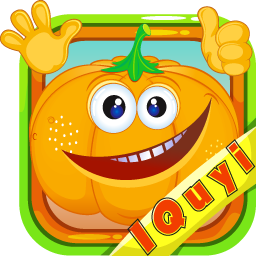#### IQuyi!

A funny math game that challenges your speed in answering math equations.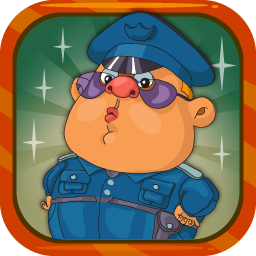#### Math Defender

Defend your planet against enemy air attack by quickly answering math equations.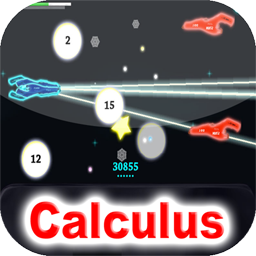#### Calculus - Math Space Battle

Control your spaceship and defeat your enemies by solving math equations.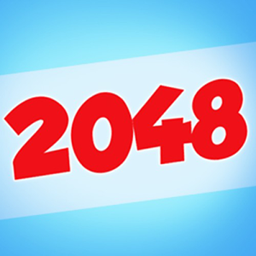#### Brain Liberty - 2048

A similar game to the famous and popular 2048 game which also rate your performance.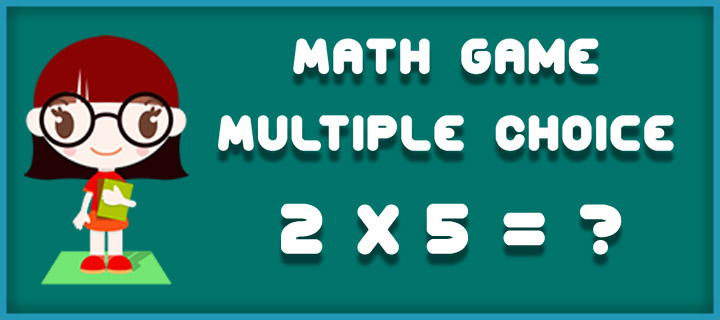#### Math Game: Multiple Choice

A multiple choice math quiz in which you have to do your best to quickly answer elementary arithmetic equations.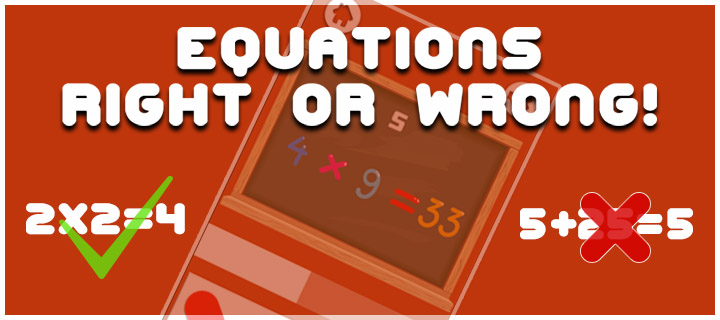#### Equations: Right or Wrong!

Do your best and try to decide which equation is right and which one is wrong as fast as you can!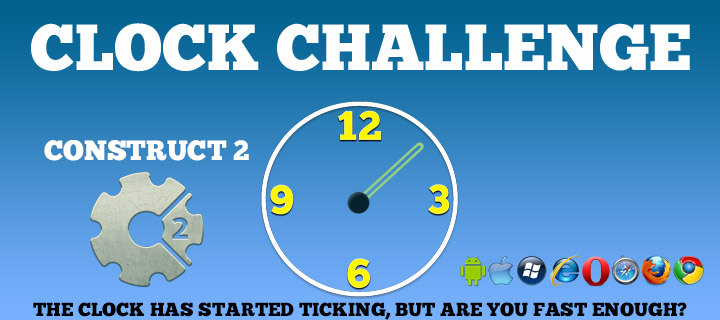#### Clock Challenege

A simple yet challenging game that tests your reaction and also teach numbers and how to read them.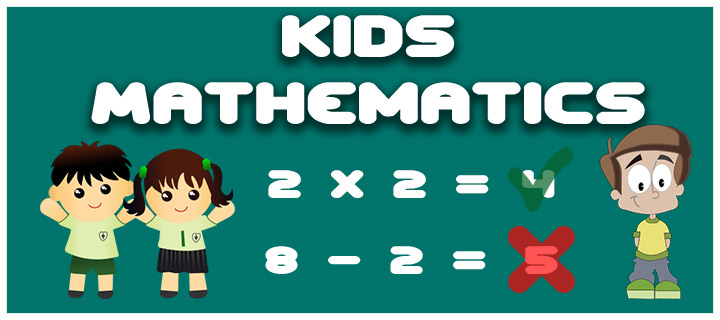#### Kids Mathematics Game

Test your mathematics skill in an intense 120 seconds quiz!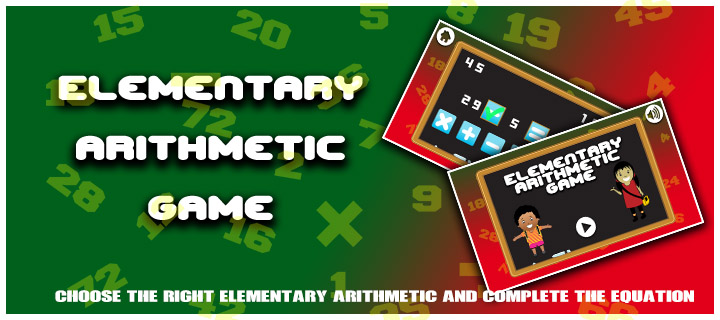#### Elementary arithmetic Game

Be quick and put the right elementary arithmetic in the equation so that it becomes a correct equation.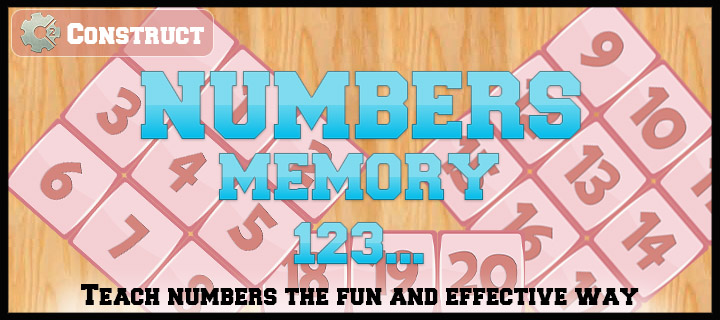#### Numbers Memory

An interesting memory game designed to teach numbers up to 20 to kids.

10 + 10 =